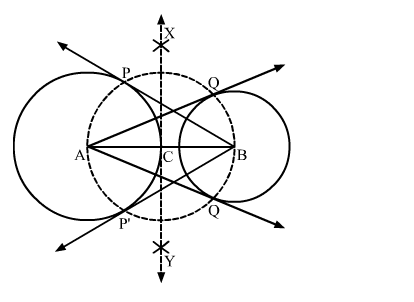# Draw a line segment AB of length 8 cm.`
Question:

Draw a line segment AB of length 8 cm. Taking A as centre, draw a circle of radius 4 cm and taking B as centre, draw another circle of radius 3 cm. Construct tangents to each circle from the centre of the other circle.

Solution:

Steps of Construction

Step 1. Draw a line segment AB = 8 cm.

Step 2. With A as centre and radius 4 cm, draw a circle.

Step 3. With B as centre and radius 3 cm, draw another circle.

Step 4. Draw the perpendicular bisector XY of AB, cutting AB at C.

Step 5. With C as centre and radius AC (or BC), draw a circle intersecting the circle with centre A at P and P'; and the circle with centre B at Q and Q'.

Step 6. Join BP and BP'. Also, join AQ and AQ'.Here, AQ and AQ' are the tangents from A to the circle with centre B. Also, BP and BP' are the tangents from B to the circle with centre A.